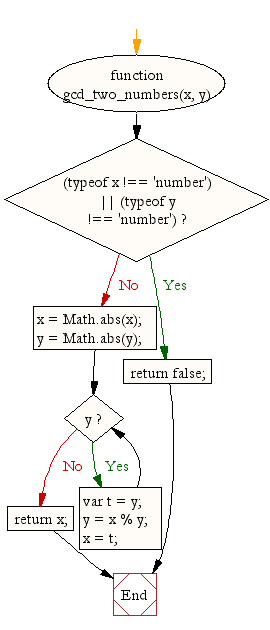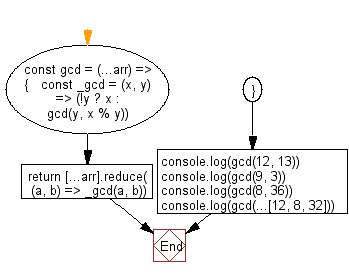# JavaScript: Greatest common divisor (gcd) of two integers

## JavaScript Math: Exercise-8 with Solution

Write a JavaScript function to get the greatest common divisor (GCD) of two integers.
Note:
According to Wikipedia - In mathematics, the greatest common divisor (gcd) of two or more integers, when at least one of them is not zero, is the largest positive integer that divides the numbers without a remainder. For example, the GCD of 8 and 12 is 4.

Test Data :
console.log(gcd_two_numbers(12, 13));
console.log(gcd_two_numbers(9, 3));
Output :
1
3

Pictorial Presentation:Sample Solution-1:

JavaScript Code:

``````function gcd_two_numbers(x, y) {
if ((typeof x !== 'number') || (typeof y !== 'number'))
return false;
x = Math.abs(x);
y = Math.abs(y);
while(y) {
var t = y;
y = x % y;
x = t;
}
return x;
}

console.log(gcd_two_numbers(12, 13));
console.log(gcd_two_numbers(9, 3));
```
```

Sample Output:

```1
3
```

Flowchart:Sample Solution-2:

Calculates the greatest common divisor between two or more numbers/arrays.

• The inner _gcd function uses recursion.
• Base case is when y equals 0. In this case, return x.
• Otherwise, return the GCD of y and the remainder of the division x/y.

JavaScript Code:

``````//Source:https://bit.ly/3hEZdCl
//gcd
const gcd = (...arr) => {
const _gcd = (x, y) => (!y ? x : gcd(y, x % y));
return [...arr].reduce((a, b) => _gcd(a, b));
};
console.log(gcd(12, 13));
console.log(gcd(9, 3));
console.log(gcd(8, 36));
console.log(gcd(...[12, 8, 32]));
```
```

Sample Output:

```1
3
4
4
```

Flowchart:Live Demo:

See the Pen javascript-math-exercise-8 by w3resource (@w3resource) on CodePen.